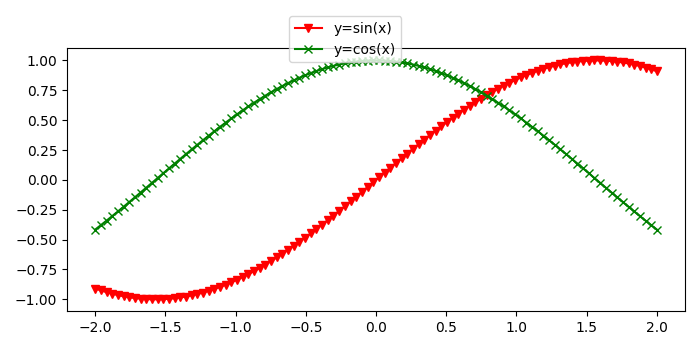# Matplotlib savefig with a legend outside the plot

To save a file with legend outside the plot, we can take the following steps −

• Create data points using numpy.

• Plot y=sin(x) curve using plot() method, with color=red, marker="v" and label y=sin(x).

• Plot y=cos(x), curve using plot() method, with color=green, marker="x" and label y=cos(x).

• To place the legend outside the plot, use bbox_to_anchor(.45, 1.15) and location="upper center".

• To save the figure, use savefig() method.

## Example

import numpy as np
from matplotlib import pyplot as plt
plt.rcParams["figure.figsize"] = [7.00, 3.50]
plt.rcParams["figure.autolayout"] = True
x = np.linspace(-2, 2, 100)
plt.plot(x, np.sin(x), c="red", marker="v", label="y=sin(x)")
plt.plot(x, np.cos(x), c="green", marker="x", label="y=cos(x)")
plt.legend(bbox_to_anchor=(.45, 1.15), loc="upper center")
plt.savefig("legend_outside.png")

## Output

When we execute this code, it will save the following plot with the name "legend_outside.png" in the current directory.